Maths Topic – Area

Myself and a number of my colleagues have been taking part in a lesson study with the PDST over the last few weeks. This involved a number of meetings with an advisor from the PDST and the members of the lesson study group (Myself and 4 colleagues). We decided on the topic of Area to use for the lesson study as I find it is a topic that children learn off the formula (length by breadth) but often have little understanding of the concept of area so we decided to use the Measures Booklet from the PDST (which you can download free of charge

Activity 1:

Firstly we did a brainstorm on everything we knew about area. It was a great way to see what the children knew already, any misconceptions they had about area too.We used a variety of objects (dominoes, dice, cards, paper, envelopes, books etc.) in the classroom to find the area of the tables/ whiteboards/ maths books etc. This ensured that the children understood the concept of area. Children covered the whiteboards/tables with these objects and then stated that the area was X dominoes etc.These were the objects we used.The area of the copy is 9 dice.

The domino problem; 44 dominoes cover the area of the copy (all in straight rows). I then took the dominoes off and began to rearrange them and showed it under the visualiser when it was half way and asked if I change the direction of the dominoes – can you estimate how many will not cover the area of the copy. The majority of children said that more or less dominoes would be needed – showing me that they did not understand the concept of area. I took a variety of different answers and then showed the fully covered copy and we counted together – the children then realised that each domino covers the same amount of area so if we use the same objects (to measure area) and the copy then the amount of objects needed should be the same.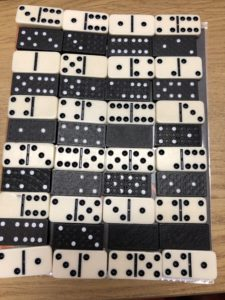Next we looked at two triangles and I asked the children to imagine that these two triangles were different lollipops. They were asked to create different combinations of the two triangles.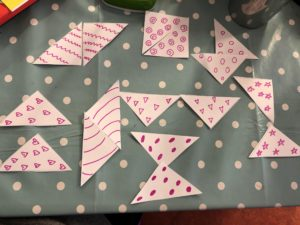I then asked which one would you like – which one is the biggest lollipop? (covers the greatest area) and the majority of children knew that they were all the same size as they were made up of 2 triangles each.

Activity 2:Estimate how many of each object would be needed to find the area of the square and which object would work best. (Children realised that the badges (circular) wouldn’t be good to find the area as they can’t tessellate. We also realised that the dominoes actually have a curved edge so they too wouldn’t be the best object to find the area of the square.

Activity 3: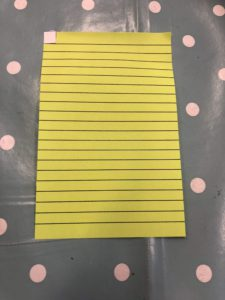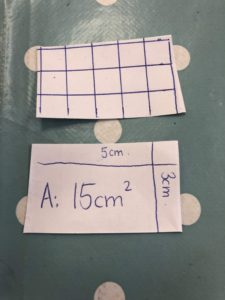We looked at what 1 squared cm actually meant and what it looked like. In the first picture the children estimated the size of the piece of paper in squared centimetres. Their estimates were initially very small so I made a squared centimetre and the children could then see what the squared centimetre looked like and they could make a more accurate estimate.

We then looked at the two pieces of paper on the right hand size and measured on using squared centimetres and the other using length by width. The children could really visualise the squared centimetres then.

We discussed the importance of using a standard unit of measurement.

The children then drew shapes covering a given area and then drew rectangles with a given length and width and calculated the area.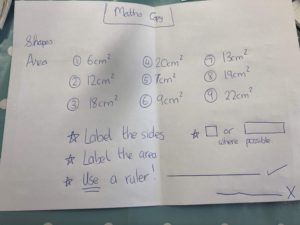Activity 4:

Estimate the area of these objects. First measure the length of the width and length and multiply to find the area.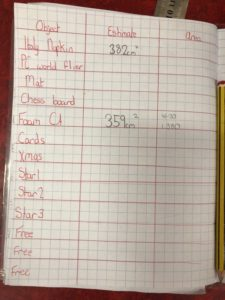The children created this grid in their copies where they wrote the object name, their estimate of the area that it would cover and the actual area. The objects used were just random things in the classroom from a PC World Leaflet, to a chess board, a Halloween mat, and washi tape stuck to the floor and also playing cards which I sellotaped to the floor to make a rectangle.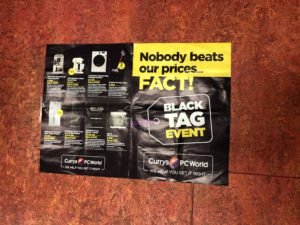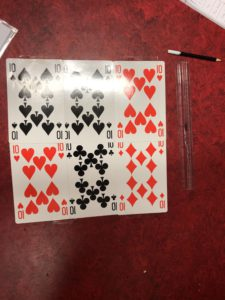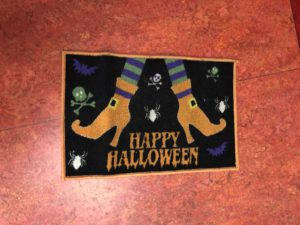The children measured the length and width of each object and multiplied them to find the area.

Activity 5:

We used Geoboards – which were a brilliant resource for teaching area.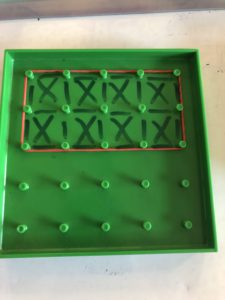Calculate the area covered by the elastic band (measurement in squares).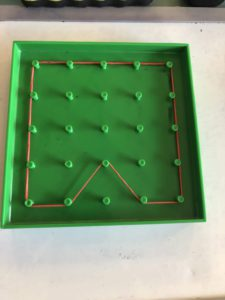We then looked at irregular shapes (as seen above)

Activity 6:

Calculate the area of irregular 2D shapes (rectangles).

First the children used the geoboards and had two elastic bands which they used to make two rectangles joined together.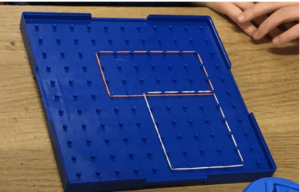We then looked at different scenarios (involving the teachers that were in the room for the lesson study).

• A teacher needs help as her dog has destroyed the back garden and the landscaper charges per metre squared.
• A teacher needs new tiles for their house. Calculate the area of the hallway.
• A teachers husband has destroyed the sitting room carpet with his muddy boots. The cleaners charger per metre squared.
• A teacher needs new wallpaper – how much will she need to buy.

The children were asked to come up with at least 1 way to find the area.

Initially the children began by divided the space into two rectangles but many groups forgot that the measurements would then change.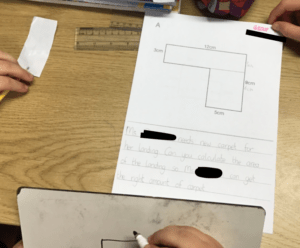Overall this was a very different approach to how I usually teach area and I have noticed a huge improvement in the children’s understanding of this concept.